# MCQs on Control Systems

##### Page 6 of 23. Go to page 1 2 3 4 5 6 7 8 9 10 11 12 13 14 15 16 17 18 19 20 21 22 23
01․ Adding a pole to a system transfer function in terms of compensator represents a --------------- compensator ?
lag

Compensators in control system are used for improving the performance specifications, i.e. transient and steady state characteristics.
1. Lead Compensator: Adding a zero to a system transfer function in terms of compensator represents a lead compensator.
2. Lag Compensator: Adding a pole to a system transfer function in terms of compensator represents a lag compensator.

02․ A linear time invariant system, initially at rest when subjected to a unit step input gave response c(t) = te-t (t ≥ 0). The transfer function of the system is
1/s(s+1)2
(s+1)2/s
s/(s+1)
s/(s+1)2

Transfer function = C(s)/R(s) Output response C(t) = te-t (t ≥ 0) C(s) = 1/(s+1)2 Given input r(t) is a step input. R(s) = 1/s Therefore, transfer function = C(s)/R(s) = S/ (S+1)2.

03․ Given the transfer function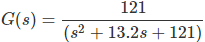of a system. Which of the following characteristics does it have?
Over damped
Critical damped
Under damped
any of the above

Given the transfer functionThe standard second order expression in terms of damping ratio (ξ) and natural frequency (ωn) is as follows.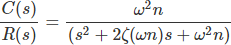Compare given transfer function with standard second order transfer function,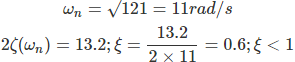Therefore given system is an under damped system.

04․ The unit impulse response of second order system is 1/6 * e-0.8t sin(0.6t). THen the natural frequency is

Impulse response = 1/6 * e-0.8t sin(0.6t) Transfer function = L(Impulse response) Transfer function = 1/6 * 0.6/((s+0.8)² + 0.6² )² = 0.1/(s² + 1.6s + 1) Therefore, ω²n = 1 Natural frequency = 1 rad/s

05․ Which of the following transfer functions represent under damped second order systems?
1/(s² + s + 1)
4/(s² + 2s + 4)
2/(s² + 2s + 2)
all of the above

The standard second order expression in terms of damping ratio (ξ) and natural frequency (ωn) is as follows. C(s)/R(s) = (ω²n)/(s² + 2ζ(ωn)s + ω²n) For under damped system : 0 < ξ < 1

06․ The open loop transfer function of a unity feedback control system is given by G(s) = k/s(s+1). If gain k is increased to infinity, then damping ratio will tend to become
infinite
0.707
unity
zero

Characteristic equation is s(s+1)+k = 0 s²+s+k = 0 The standard second order expression in terms of damping ratio (ξ) and natural frequency (ωn) is as follows. C(s)/R(s) = (ω²n)/(s² + 2ζ(ωn)s + ω²n) Compare to second order characteristic equation, 2ζ(ωn) = 1 ωn = √k Damping ratio ξ = 1/(2√k) As k → ∞, damping ratio will become zero.

07․ Consider a network function H(s) = 2(s+3)/{(s+2)(s+4)}. What is the steady state response due to step input?
4/3
1/2
1
3/4

Steady state value can be obtained by applying final value theorem to the function. Lims→0 s C(s) = Lims→0 s R(s)H(s) Where, C(s) = Steady state value R(s) = Input H(s) = Network response Steady state value = Lims→0 s *2(s+3)/((s+2)(s+4))*1/s = 3/4

08․ The system function N(s) = V(s)/I(s) = (s+3)/(4s+5). The system is initially at rest. If the excitation i(t) is a unit step, which of the following is the initial value?
0
3/5
1/4
3/9

Initial value can be obtained by applying initial value theorem to the function. Lims→0 s C(s) = Lims→∞ s R(s)H(s) Where, C(s) = Steady state value R(s) = Input H(s) = Network response Initial value = Lims→∞ s*(s+3)/(4s+5)*1/s = 1/4

09․ The open loop transfer function for unity feedback system is given by 5(1+0.1s) / (s(1+5s)(1+20s)) Find the steady state error for a step input of magnitude 10 is equal to
zero.
2.
infinite.
5.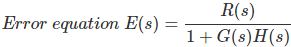Applying final value theorem, Steady state error = Lts→0 S E(s) Steady state error for different types and different inputs as follows: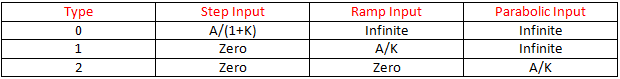10․ The input-output relationship of a linear time invariant continuous time system is given by r(t) = d²c(t)/dt² + 3 dc(t)/dt + 2 c(t) Where r(t) and c(t) are input and output respectively. What is the transfer function of the system equal to?
1/(s² + s + 2)
1/(s² + 3s + 2)
2/(s² + 3s + 2)
2/(s² + s + 2)

Transfer function = C(s)/R(s) Given that, r(t) = d²c(t)/dt² + 3 dc(t)/dt + 2 c(t) By taking Laplace transforms on both sides, C(s)/R(s) = 1/(s² + 3s + 2)

<<<45678>>>